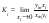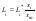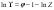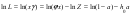• Submissions# Thermodynamics of the Separation of Inorganic Substances During Crystallization from Electrolyte Solutions

Allakhverdov GR*

State Scientific-Research Institute of Chemical Reagents and High Purity Chemical Substances- National Research Centre, Kurchatov’s Institute, Russia

*Corresponding author: Allakhverdov GR, State Scientific-Research Institute of Chemical Reagents and High Purity Chemical Substances–National Research Centre, Kurchatov’s Institute, Russia

Submission: May 31, 2019;Published: July 09, 2019

DOI: 10.31031/AMMS.2019.03.000557ISSN 2578-0255
Volume3 Issue2

#### Abstract

A new method for calculating the separation factor in the processes of crystallization of the inorganic substances from aqueous solutions is proposed. The calculated values of the separation factor are in good agreement with the experimental data. As the initial data for the calculation are used the solvent activity data in binary saturated solutions of the impurity and major components.

1. Keywords: Separation factor; Activity of component; Isopiestic solutions

#### Introduction

When crystallization from solution is used to obtain pure inorganic substances, the degree of purification of the major component in the most important range of trace impurity concentration can be characterized by the limiting separation factor. This parameter Ki can be represented is the limit of the rate of the component concentrations in phases coexisting in equilibrium:where the subscript i and m refer to the impurity and major component, respectively; y is the mole fraction of the impurity or major component in the solid phase, and x is the relative concentration, which proportional molality of the component in the liquid phase, x=νm/W, 𝜈-stoichiometric coefficient of the electrolyte, m–molality, W–number of moles of the solvent per kilogram (W=55,51 for water). This paper presents a theoretical estimate of characteristics of interaction between components in mixed electrolyte solutions and calculation of separation factors for the purification of inorganic substances by crystallization.

#### Theory

According to the Nernst distribution law, under phase equilibrium conditions the thermodynamics separation factor Ki0 can be defined aswhere S and L the activities of components in the solid and liquid phases, respectively. Ki0 retains its meaning at any relationship between components, including the limiting case of pure components. Taking the activity of pure components in the solid phase to be unity, the Eq.(2) can be represented as the followingwhere Li0 and Lm0 are the activity of the impurity and major component in their saturated binary solutions. Comparing Eqs.(2) and (3) and taking into account that, at trace impurity levels, Lm⤑Lm0 for xi⤑0, we can write following relation:where Li is the activity of the impurity component in the mixed solution. For xi⤑0, to a good approximation this parameter can be represented in the form:where Li* is the activity of the impurity component in its isopiestic binary solution having the same solvent vapor pressure as the mixed solution. Combining Eqs.(4) and (5) and substitution S=yf, where f is the activity coefficient of a component in the solid phase, we obtainComparing Eqs (1) and (6) and taking account that, under the condition in question, we can take fm=1, we finally havewhere fi is the activity coefficient of the impurity component in the solid phase. Relation (7) is similar to the Ratner equation , with the distinction that liquid-phase interaction is represented here in explicit form as the ratio of the impurity activities in different solutions.

Thus, we are led to the important conclusion that Ki is fully determined by the properties of the impurity component in the host component: in a solid phase, by fi , and in a liquid phase, as the ratio of the activity in its saturated solution to the activity in a binary solution isopiestic to a mixed solution or, in the case under consideration (for xi⤑0), isopiestic to saturated solution of the major component. The activity coefficient of the impurity component fi can be calculated using known methods for estimating the interaction of solid solution components [2-4], so below we consider the possibility of theoretical calculation of the ratio (Li0)/(Li* ). According to statistical model of electrolyte solutions  the relationship between the activity coefficient of the solute and the osmotic coefficient of the solution can be represented in the formwhere Z is the partition function of the solution. By potentiating this relation and limiting it to the linear representation of the exponent, we haveIn concentrated solutions, the main contribution to the partition function is made by the process of hydration of electrolytes, so we can use the total ratiowhere h is the hydration number of electrolyte, a is the activity of solvent. Using the decomposition the partition function Z in Taylor series by the solvent activity degrees and being limited by the linear term, we obtain approximationwhere h0 is the hydrate number of the electrolyte in an infinitely diluted solution. Using approximationand combining Eqs.(9) and (11), activity of component L can be represented in the form:Using Eq.(13), the ratio of the electrolyte activities in a binary solution at different water activities can be determined asand Eq.(7) can be transformed to its final formwhere ai and am are the solvent activities in binary saturated solution of the impurity and major component, respectively.

#### Discussion

Thus, Eq.(15) allows separation factor to be calculated from available data for binary solutions of a major component and impurity. The (table 1) show compliance of the calculated and experimental data on the example of aqueous solutions of potassium salts with various impurity. The impurity component is written after the major component. The experimental Ki and am ,ai values are borrowed from [6-9]. Consider systems where interaction between their components can be neglected and fi can be taken to be unity. Given the definition of solvent activity, the separation factor can then be represented aswhere P0 is the vapor pressure of the pure solvent, and Pi and Pm are the vapor pressures over binary saturated solutions of the impurity and major component, respectively. Therefore, the equilibrium separation factor increases with decreasing vapor pressure over the binary saturated impurity solution. This result can be used in the general case as a first approximation for estimating a priori the purity of a major component in terms of various impurities.

Table 1:Separation factor for the crystallization of potassium salts from aqueous solution at 298 K.#### Conclusion

A new method for calculating the separation factor in the processes of crystallization of the inorganic substances from aqueous solutions is proposed. Moreover, only data of binary solutions of the major and impurity components (solvent activity in saturated solutions, or vapor pressure over solutions) can be used as the initial data for the calculation, without using experimental data for mixed solutions. The calculated values of the separation factor are in good agreement with the experimental data.

2. Wasastjerna JA (1950) On the theory of the heat formation of solid solutions. Soc Sci Fenn Comm Phys-math 15(12): 1-14.

3. Hietala J (1963) Alkali halide solid solution II. Annal Acad Sci Fenn A VI Physica 122: p.32 .

4. Allakhverdov GR (1980) Calculation of the thermodynamics functions of formation of solid solutions. Zh Fis Khim 54(3): 617-621.

5. Allakhverdov GR (2008) Thermodynamics of electrolyte solutions. Doklady Physica 53(8): 420-424.

6. Allakhverdov GR, Nevinchan OM, Sandu RA (2013) Cocrystallization of inorganic substances with nonisomorphous impurities. Khim Prom st Segodnya 12: 3-5.

7. Melikhov IV, Merkulova MS (1975) Cocrystallization. Moscow, Russia.

8. Robinson RA, Stokes RH (1955) Electrolyte solutions. London.

9. Mikulin GI (ed.) (1968) Current issues in the physical chemistry of electrolytes solutions. Leningrad, Russia.

﻿

We at Crimson Publishing are a group of people with a combined passion for science and research, who wants to bring to the world a unified platform where all scientific know-how is available read more...